Courses

# Test: Measurement of Pressure, pH & Viscosity- 2

## 25 Questions MCQ Test Sensor & Industrial Instrumentation | Test: Measurement of Pressure, pH & Viscosity- 2

Description
This mock test of Test: Measurement of Pressure, pH & Viscosity- 2 for GATE helps you for every GATE entrance exam. This contains 25 Multiple Choice Questions for GATE Test: Measurement of Pressure, pH & Viscosity- 2 (mcq) to study with solutions a complete question bank. The solved questions answers in this Test: Measurement of Pressure, pH & Viscosity- 2 quiz give you a good mix of easy questions and tough questions. GATE students definitely take this Test: Measurement of Pressure, pH & Viscosity- 2 exercise for a better result in the exam. You can find other Test: Measurement of Pressure, pH & Viscosity- 2 extra questions, long questions & short questions for GATE on EduRev as well by searching above.
QUESTION: 1

### What influences viscosity?

Solution: Along with the shear rate, the temperature really is the dominating influence. The higher the temperature is, the lower a substance’s viscosity is. Consequently, decreasing temperature causes an increase in viscosity. For some fluids, a decrease of 1°C already causes a 10 % increase in viscosity.
QUESTION: 2

### A capillary tube is 30 mm long and 1 mm bore. The heat required to produce a flow rate of 8 mm3/s is 30 mm. The fluid density is 800 kg/m3. Calculate the dynamic viscosity of the oil.

Solution: Rearranging Poiseuille’s equation we get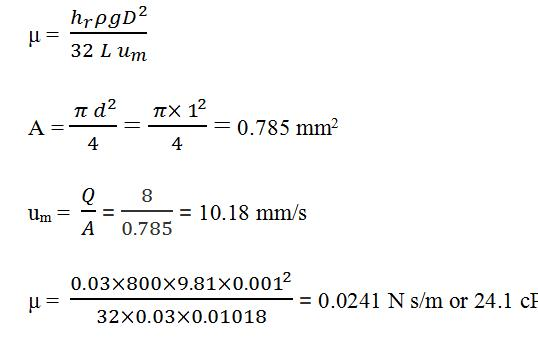QUESTION: 3

### Taking question 7 into consideration, and calculate the kinematic viscosity of the oil.

Solution: v = μ/ρ = 0.0241/800 = 30.11 × 10-6 m2/s or 30.11 cSt.
QUESTION: 4

Which of the following factors do not determine the Blood viscosity?

Solution: Five primary factors determine blood viscosity. These include hematocrit, erythrocyte deformability, plasma viscosity, erythrocyte aggregation, and temperature. Hematocrit is the most obvious determinant of WBV. A higher percentage of red blood cells (RBCs) results in thicker blood.
QUESTION: 5

At the bottom of a uniform cylindrical vessel of 30 cm height a horizontal capillary tube of 2mm inner diameter and 10 cm length is connected to discharge the liquid. Calculate the rate of discharge of the liquid if the vessel contains water of viscosity 0.01 poise to its 2/3 capacity. Take the density of water as 1 gm/cc.

Solution: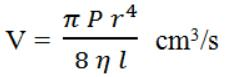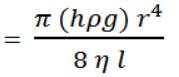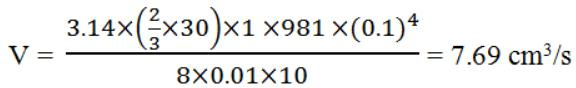QUESTION: 6

Taking question 10 into consideration, and Calculate the rate of discharge of the liquid if the vessel contains oil of viscosity 0.84 poise to its 2/3 capacity. Take the density of oil as 0.9 gm/cc.

Solution: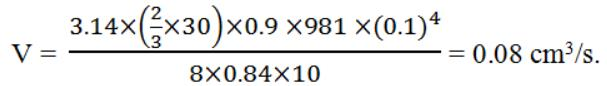QUESTION: 7

What is the pH of the seawater?

Solution: Solutions with low pH are acidic and solutions with high pH are basic (also known as alkaline). Prior to the Industrial Revolution, the average ocean pH was about 8.2. Today, the average ocean pH is about 8.1.
QUESTION: 8

Operation of McLeod gauge used for low-pressure measurement is based on the principle of

Solution: Mcleod Gauge works on the principle of Boyle's Law. Boyle's Law states that if the temperature and amount of gas remain unchanged, the absolute pressure exerted by a given mass of gas is inversely proportional to the volume it occupies.
QUESTION: 9

Which of the following is not a type of pressure sensing element?

Solution: The main types of sensing elements are Bourdon tubes, diaphragms, capsules, and bellows. The Bourdon tube is a sealed tube that deflects in response to applied pressure.
QUESTION: 10

Piezometer is used to measure the pressure of a

Solution: Piezometer is used to measure underground water pressure. It converts water pressure to a frequency signal via a diaphragm and a tensioned steel wire. A change in pressure on the diaphragm causes a change in tension of the wire.
QUESTION: 11

A Piezometer is used to measure

Solution: A piezometer is either a device used to measure liquid pressure in a system by measuring the height to which a column of the liquid rises against gravity or a device that measures the pressure (more precisely, the piezometric head) of groundwater at a specific point.
QUESTION: 12

A manometer is used to measure the pressure of a

Solution: A manometer can be designed to directly measure absolute pressure. The manometer in Figure 5 measures the pressure compared to zero absolute pressure in a sealed leg above a mercury column. The most common form of this manometer is the conventional mercury barometer used to measure atmospheric pressure.
QUESTION: 13

A manometer is used to measure the pressure of a

Solution: Manometers are devices in which columns of a suitable liquid are used to measure the difference in pressure between two points or between a certain point and the atmosphere. The manometer is needed for measuring large gauge pressures.
QUESTION: 14

A differential manometer is used to measure the pressure of a

Solution: Differential manometers are used to measure the difference of pressures between two points in a pipe or in two different pipes. There are two types of differential manometers. It is used to measure pressure difference at two points in a pipe or between two pipes at different levels.
QUESTION: 15

A differential manometer is used to measure the pressure difference between two points in a flowing

Solution: Differential manometers are used to measure the difference of pressures between two points in a pipe or in two different pipes. There are two types of differential manometers. It is used to measure pressure difference at two points in a pipe or between two pipes at different levels.
QUESTION: 16

A U-tube manometer is used to measure the pressure of a

Solution: Pressure measuring devices using liquid columns in vertical or inclined tubes are called manometers. One of the most common is the water-filled u-tube manometer used to measure pressure difference in pitot or orifices located in the airflow in air handling or ventilation system.
QUESTION: 17

A inverted tube manometer is used to measure the pressure difference between two points of a

Solution: An inverted U-tube manometer is used to measure the pressure difference between two pipes A and B, as shown in the figure. Pipe A is carrying oil (specific gravity = 0.8) and pipe B is carrying water. The densities of air and water are 1.16 kg/ m3 and 1000 kg/m3, respectively.
QUESTION: 18

A Bourdon tube pressure gauge measures

Solution: Bourdon tube pressure gauges are used for the measurement of relative pressures from 0.6 ... 7,000 bar. They are classified as mechanical pressure measuring instruments and thus operate without any electrical power. Bourdon tubes are radially formed tubes with an oval cross-section.
QUESTION: 19

Bourdon tubes are generally made of

Solution: The Bourdon tube is an almost rectangular or elliptical cross-section tube made from materials such as stainless steel or phosphor bronze.
QUESTION: 20

Which of the following is a mechanical gauge?

Solution: Mechanical gauges are instruments that measure pressure, dimensions, levels, etc. They can be mechanical or electro-mechanical devices and offer displays ranging from direct-reading rules to digital LCDs. Gauges that measure pressure are classified as analog or digital depending on their readouts.
QUESTION: 21

The simplest form of manometer used for measuring moderate pressures is

Solution: Piezometer is one of the simplest forms of manometers. It can be used for measuring moderate pressures of liquids. The setup of piezometer consists of a glass tube, inserted in the wall of a vessel or of a pipe. The tube extends vertically upward to such a height that liquid can freely rise in it without overflowing.
QUESTION: 22

Inclined single column manometer is useful for which of the pressure

Solution: The inclined manometer is essential for retaining the most accurate pressure levels for industrial gas applications. A low-pressure industrial gas system may be used to heat or cool manufacturing processes. A small blockage within the gas system can be detected with an inclined manometer and corrected.
QUESTION: 23

pH meters can be considered as voltage sources with which of the following internal resistances

Solution: pH meters can be considered as voltage sources with very high internal resistance. In order to eliminate errors, no current should flow from the source.
QUESTION: 24

The electrodes used in pH measurement have which of the following internal resistances?

Solution: The electrodes used in pH measurement have very high internal resistance. It is of the order of 1000M ohm.
QUESTION: 25

Which of the following is not a failure in pH meters?

Solution: Defective calibration is not a failure in pH meters. Failure occurs due to defective electrodes, defective input circuitry, and defective electronic circuitry.